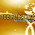## Sunday, 10 March 2013

### JAVA PROGRAM: TO FIND SUM OF DIGITS OF A NUMBER

CODING OF ACTION BUTTON(jButton1)
int num,temp,sum = 0;
num=Integer.parseInt(jTextField1.getText());
while(num>0)
{
temp=num%10;
sum+=temp;
num=num/10;
}
jTextField2.setText(" "+sum);

CODING OF CLEAR BUTTON(jButton2)
jTextField1.setText(" ");
jTextField2.setText(" ");

CODING OF EXIT BUTTON(jButton3)
System.exit(0);CODINGRUNNING

We have used NetBeans IDE 7.2.1. To download it visit www.netbeans.org

For any query or suggestion please comment below...

#### 2 comments:

1.thanks for the code!
can you just explain it to me roughly

1.Using "temp=num%10;" we got the remainder i.e. last digit of number "num". Then we added it to "sum" ("sum" is 0 at starting) using "sum+=temp;". Now we will remove last digit of number by "num=num/10;" . We will repeat this until "num" is 0.

Hope you understood. If you have any doubt please feel free to ask.